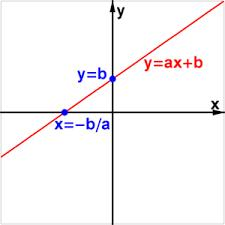# Equation 4300

Solve the equation and perform the test:
1- (x-x/7-1/7) = 7-9x/2 +5/2

x =  2.2941

### Step-by-step explanation:Did you find an error or inaccuracy? Feel free to write us. Thank you!

Tips for related online calculators
Do you have a linear equation or system of equations and looking for its solution? Or do you have a quadratic equation?